Students can Download Tamil Nadu 12th Computer Science Model Question Paper 2 English Medium Pdf, Tamil Nadu 12th Computer Science Model Question Papers helps you to revise the complete Tamilnadu State Board New Syllabus, helps students complete homework assignments and to score high marks in board exams.

## TN State Board 12th Computer Sceince Model Question Paper 2 English Medium

General Instructions:

1. The question paper comprises of four parts.
2. You are to attempt all the parts. An internal choice of questions is provided wherever applicable.
3. All questions of Part I, II, III and IV are to be attempted separately.
4. Question numbers 1 to 15 in Part I are Multiple Choice Questions of one mark each.
These are to be answered by choosing the most suitable answer from the given four alternatives and writing the option code and the corresponding answer
5. Question numbers 16 to 24 in Part II are two-mark questions. These are to be answered in about one or two sentences.
6. Question numbers 25 to 33 in Part III are three-mark questions. These are to be answered in about three to five short sentences.
7. Question numbers 34 to 38 in Part IV are five-mark questions. These are to be answered in detail Draw diagrams wherever necessary.

Time: 3 Hours
Maximum Marks: 70

PART – 1

Choose the correct answer. Answer all the questions: [15 × 1 = 15]

Question 1.
Which of the following defines what an object can do?
(a) OS
(b) Computer
(c) Interface
(d) Interpreter
(c) InterfaceQuestion 2.
Identify the constructor from the following?
(a) City=makecity(name, lat, Ion)
(b) Getname (city)
(c) Getlat (city)
(d) Getlon (city)
(a) City=makecity(name, lat, Ion)

Question 3.
Which of the following security technique that regulates who can use resources in a computing environment?
(b) Authentication
(c) Access control
(d) Certification
(c) Access controlQuestion 4.
………………….. is an expression of algorithm in a programming language.
(a) Program
(b) Language
(c) Module
(d) Memory
(a) Program

Question 5.
In selection sort, there will be ……………. exchange for every pass through the list.
(a) 0
(b) 1
(c) n
(d) n2
(b) 1

Question 6.
………….. function is the alternative for bin ( ) function.
(a) Ord ( )
(b) Format ( )
(c) Binary ( )
(d) Bin ( )
(b) Format ( )Question 7.
Which command is used to remove the entire string variable?
(a) Remove
(b) Del
(c) Delete
(d) Strike
(b) Del

Question 8.
Functions defined inside a class ………………..
(a) Functions
(b) Module
(c) Methods
(d) Section
(c) MethodsQuestion 9.
In which database systems, transaction management is efficient?
(a) DBMS
(b) RDBMS
(c) ERDMS
(d) DMBS
(b) RDBMS

Question 10.
When the command to run a python program is given to interpreter, code at ……………… indentation is executed ………………..
(a) level 0
(b) level 1
(c) level 2
(d) level 3
(a) level 0Question 11.
Which has a native library for sqlite?
(a) C
(b) C++
(c) Java
(d) Python
(d) Python

Question 12.
Which table holds the key information about the database tables?
(a) Page
(b) Select
(c) Primary
(d) Master
(d) Master

Question 13.
Pick the odd one out
(a) Dashboard
(b) Live graph
(c) Barplot
(d) Histogram
(c) BarplotQuestion 14.
Which function shows the percentage value in pie chart?
(a) Percent
(b) Percentage
(c) Slice
(d) Autopct
(d) Autopct

Question 15.
Which of the following module is provided by Python to do several operations on the CSV files?
(a) Py
(b) Xls
(c) Csv
(d) Os
(d) Os

PART – II

Answer any six questions. Question No. 21 is compulsory. [6 × 2 = 12]

Question 16.
Write note on Grant and Revoke command?
Grant: Grants permission to one or more users to perform specific tasks.
Revoke: Withdraws the access permission given by the GRANT statement.Question 17.
Name some clauses in SQL?

1. DISTINCT
2. WHERE
3. GROUP BY
4. ORDER BY
5. HAVING

Question 18.
Define subroutine?
Subroutines are the basic building blocks of computer programs. Subroutines are small sections of code that are used to perform a particular task that can be used repeatedly. In Programming languages these subroutines are called as Functions.Question 19.
Write note on modules?
A module is a part of a program. Programs are composed of one or more independently developed modules. A single module can contain one or several statements closely related each other. Modules work perfectly on individual level and can be integrated with other modules.

Question 20.
Write note on Exponent data?
An Exponent data contains decimal digit part, decimal point, exponent part followed by one or more digits.

Question 21.
Write a program to check if year is leap or not?
n=int(input(“Enter any year”1)) if(n % 4 == 0):
print “Leap Year”
else:
print “Not a Leap Year”Question 22.
Write the syntax of if-else statements?
Syntax:
if:
statements-block 1 else:
statements-block 2

Question 23.
Write note on list comprehension?
List comprehension is a simplest way of creating sequence of elements that satisfy a certain condition.
Syntax:
List = [expression for variable in range]Question 24.
What is the purpose of Destructor?
Destructor is also a special method gets executed automatically when an object exit from the scope. It is just opposite to constructor. In Python, del ( ) method is used as destructor.

PART – III

Answer any six questions. Question No. 29 is compulsory. [6 × 3 = 18]

Question 25.
What are the two phases in the Analysis of algorithms and performance evaluation?
(a) A Priori estimates:
This is a theoretical performance analysis of an algorithm. Efficiency of an algorithm is measured by assuming the external factors.

(b) A Posteriori testing:
This is called performance measurement. In this analysis, actual statistics like running time and required for the algorithm executions are collected.Question 26.
Write note on Arithmetic operators?
An arithmetic operator is a mathematical operator that takes two operands and performs a calculation on them. They are used for simple arithmetic. Most computer languages contain a set of such operators that can be used within equations to perform different types of sequential calculations.

Question 27.
In Python, comments begin with hash symbol (#). The lines that begins with # are considered as comments and ignored by the Python interpreter. Comments may be single line or no multi-lines. The multiline comments should be enclosed within a set of:-
# as given below.
# It is Single line Comment
# It is multiline comment
which contains more than one line #Question 28.
Give the flowchart for ‘for loop’ execution?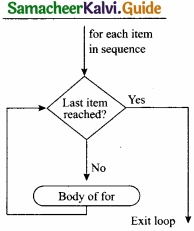Question 29.
What is the output of the following snippet?
i=1
while True:
if i%3 =0:
break
print(i,end=”)
i +=1 Output:
12Question 30.
Give the syntax for break, continue, pass?
Syntax:
break

Syntax:
continue

Syntax:
pass

Question 31.
Fill the Table with appropriate values?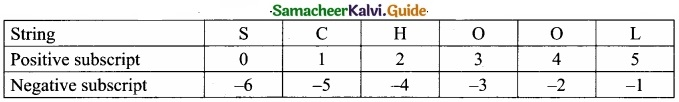Question 32.
Give the 3 different syntax formats for deleting the elements from a list?
Syntax:
del List [index of an element]
# to delete a particular element
del List [index from: index to]
# to delete multiple elements del List
# to delete entire listQuestion 33.
What is the output of the following program?
class Sample:
_num=10
def disp(self):
print(self._num)
S=Sample( )
S.disp( )
print(S._num)
Output:
Error Sample has no attribute S._num
10

PART – IV

Answer all the questions. [5 × 5 = 25]

Question 34(a).
Describe in detail the procedure script mode programming?
Basically, a script is a text file containing the Python statements. Python Scripts are reusable code. Once the script is created, it can be executed again and again without retyping. The Scripts are editable.

Creating Scripts in Python:
(I) Choose File —> New File or press Ctrl + N in Python shell window.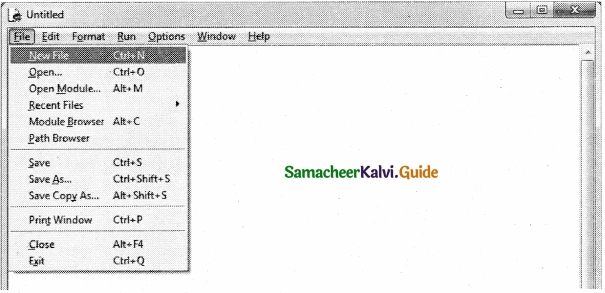(II) An untitled blank script text editor will be displayed on screen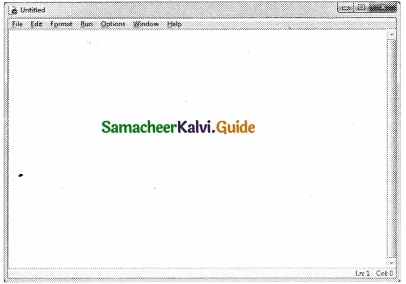(III) Type the following code in Script editor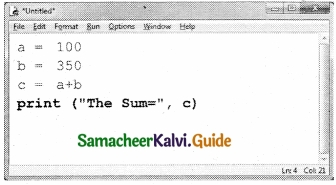a =100
b = 350
c = a + b
print (“The Sum=”, c)

Saving Python Script:

(I) Choose File → Save or Press Ctrl + S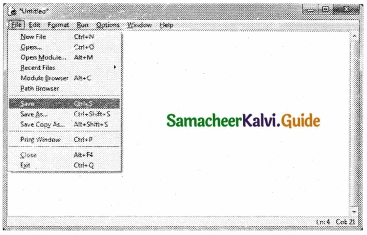(II) Now, Save as dialog box appears on the screen(III) In the Save As dialog box, select the location where you want to save your Python code, and type the file name in File Name box. Python files are by default saved with extension .py. Thus, while creating Python scripts using Python Script editor, no need to specify the file extension.

(IV) Finally, click Save button to save your Python script.

Executing Python Script:-
(I) Choose Run → Run Module or Press F5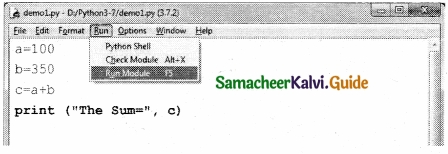(II) If your code has any error, it will be shown in red color in the IDLE window, and Python describes the type of error occurred. To correct the errors, go back to Script editor, make corrections, save the file using Ctrl + S or File → Save and execute it again.

(III) For all error free code, the output will appear in the IDLE window of python[OR]

(b) Explain data types in python?
Python Data types:
All data values in Python are objects and each object or value has type. Python has Built-in or Fundamental data types such as Number, String, Boolean, tuples, lists and dictionaries.

Number Data type:
The built-in number objects in Python supports integers, floating point numbers and complex numbers.

Integer Data can be decimal, octal or hexadecimal. Octal integer use O (both upper and lower case) to denote octal digits and hexadecimal integer use OX (both upper and lower case) and L (only upper case) to denote long integer.

Example :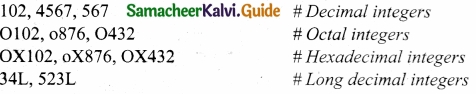A floating point data is represented by a sequence of decimal digits that includes a decimal point. An Exponent data contains decimal digit part, decimal point, exponent part followed by one or more digits.

Example :Complex number is made up of two floating point values, one each for the real and imaginary parts.

Boolean Data type:
A Boolean data can have any of the two values: True or False.

Example :
Bool_var1=True
Bool_var2=False

String Data type:
String data can be enclosed with single quote or double quote or triple quote.

Example:
Char_data = ‘A’
String_data= “Computer Science”
Multiline_data= “““String data can be enclosed with single quote or double quote or triple quote.”””

Question 35(a).
Write a program to calculate the sum of numbers from 1 to 100?
n = 100 sum = 0
for counter in range( 1 ,n+1):
sum = sum + counter
print(“Sum of 1 until %d: %d” % (n,sum))

Output:
Sum of 1 until 100: 5050

[OR]

(b) Write a program to replace a string with another string without replace ( )?
s1 = input(“Enter the-string to be replaced : “)
s2 = input(“Enter the string to replace with “)
s1 = s2
print(“Replaced string is “, si)

Output:
Enter the string to be replaced: Computer
Enter the string to replace with: repcomputer
Replaced string is repcomputer

Question 36(a).
Write a program using class to accept 3 sides of a triangle and print its area?
class Tr:
def init (self, a, b, c):
self.a = float(a)
self.b = float(b)
self.c = float(c)
def area(self):
s = (self.a + self.b + self.c)/2
return((s*(s-self.a) * (s-self.b) * (s-self.c) ** 0.5))
a = input(“Enter side 1:”)
b = input(“Enter side2:”)
c = input(“Enter side3:”)
ans=Tr(a,b,c)
print(ans.area())

Output:
Enter side 1 : 3 Enter side 2:4 Enter side 3 : 5

[OR]

(b) Explain different types of DBMS users?
Database Administrator or DBA is the one who manages the complete database management system. DBA takes care of the security of the DBMS, managing the license keys, managing user accounts and access etc.

(II) Application Programmers or Software Developers:
This user group is involved in developing and designing the parts of DBMS.

(III) End User:
End users are the one who store, retrieve, update and delete data.

(IV) Database designers:
Are responsible for identifying the data to be stored in the database for choosing appropriate structures to represent and store the data.Question 37 (a).
Explain various components of DBMS?
The Database Management System can be divided into five major components as follows:

1. Hardware
2. Software
3. Data
4. Procedures/Methods
5. Database Access Languages

1. Hardware:
The computer, hard disk, I/O channels for data, and any other physical component involved in storage of data

2. Software:
This main component is a program that controls everything. The DBMS software is capable of understanding the Database Access Languages and interprets into database commands for execution.

3. Data:
It is that resource for which DBMS is designed. DBMS creation is to store and utilize data.

4. Procedures/Methods:
They are general instructions to use a database management system such as installation of DBMS, manage databases to take backups, report generation, etc.

5. DataBase Access Languages:
They are the languages used to write commands to access, insert, update and delete data stored in any database.[OR]

(b) Differentiate IN and NOTIN in SQL using sample commands? IN Keyword?
The IN keyword is used to specify a list of values which must be matched with the record values. In other words it is used to compare a column with more than one value. It is similar to an OR condition.

For example :
SELECT Admno, Name, Place FROM Student WHERE Place IN (“Chennai”, “Delhi”);

 Adm No Name Place 100 Ashish Chennai 101 Adarsh Delhi 103 Ayush Delhi 104 Abinandh Chennai 105 Revathi Chennai 107 Hema Chennai

NOT IN:
The NOT IN keyword displays only those records that do not match in the list.

For example:
SELECT Admno, Name, Place FROM Student WHERE Place NOT IN (“Chennai”, “Delhi”); will display students only from places other than “Chennai” and “Delhi”.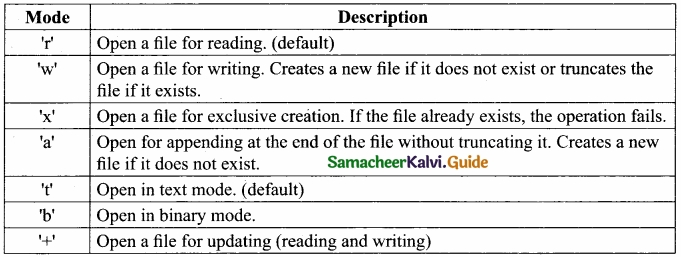Question 38 (a).
Explain various python file modes?

 Adm No Name Place 102 Akshith Bangalore 106 Devika Bangalore

[OR]

(b) Explain getopt ( ) in python?
Python getopt module:
The getopt module of Python helps you to parse (split) command-line options and arguments. This module provides two functions to enable command-line argument parsing, getopt.getopt method

This method parses command-line options and parameter list. Following is the syntax for this method
=getopt.getopt( argv, options, {long_options])
Here is the detail of the parameters –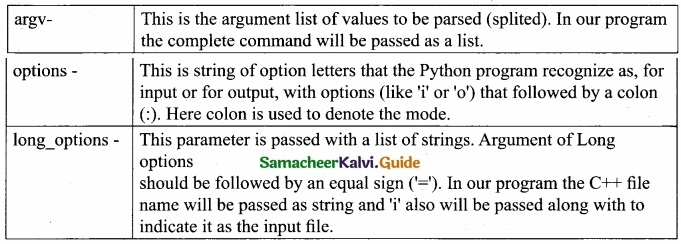getopt( ) method returns value consisting of two elements. Each of these values are stored separately in two different list (arrays) opts and args .Opts contains list of splitted strings like mode, path and args contains any string if at all not splitted because of wrong path or mode, args will be an empty array if there is no error in splitting strings by getopt( ). For example The Python code which is going to execute the C++ file p4 in command line will have the getopt Q method like the following one. opts, args = getopt.getopt (argv, “i:”,[‘ifile=’])>>>print(args)
[ ]
Some more command for wrapping C++ code

if _name _==’_main_’;
main(sys.argv[l:])
_name_(A Special variable) in Python

Since there is no main( ) function in Python, when the command to run a Python program is given to the interpreter, the code that is at level 0 indentation is to be executed. However, before doing that, interpreter will define a few special variables. _name_ is one such special variable which by default stores the name of the file.

If the source file is executed as the main program, the interpreter sets the _name_ variable to have a value as ” main ” name is a built-in variable which evaluates to the name of the current module. Thus it can be used to check whether the current script is being run on its own.

For example consider the following
if_name_==’_main_’:
main(sys.argv[1:])
if the command line Python program itself is going to execute first, then _main_ contains the name of that Python program and the Python special variable _name_ also contain the Python program name. If the condition is true it calls the main which is passed with C++ file as argument.